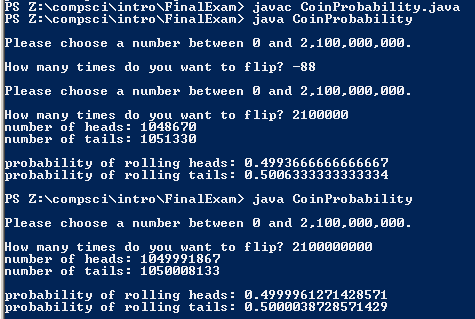# FinalExam: CoinProbability

## Code

```      /// Name: Jason Kim
/// Period: 7
/// Program Name: CoinProbability
/// File Name: CoinProbability.java
/// Date Finished:1/21/16

import java.util.Scanner;
import java.util.Random;

public class CoinProbability
{
public static void main(String[] args)
{
Scanner keyboard = new Scanner(System.in);
Random r = new Random();
int flipCounter = 0, tails = 0, heads = 0, flip;//tails: number of tails, heads = number of heads,flip: the number of flips the user wants to do

do
{
System.out.println("\nPlease choose a number between 0 and 2,100,000,000.");
System.out.print("\nHow many times do you want to flip? ");
flip = keyboard.nextInt();
}while( flip> 2100000000 || flip < 1);//checks if the number is between 1 and 2100000000

while( flipCounter < flip)
{
int side = r.nextInt(2);
//0 = tail, 1 = head
if( side == 0)
{
tails ++;
}
else
{
}
flipCounter++;
}

double probOfTails = (double)tails / flip;

System.out.println("number of tails: " + tails);